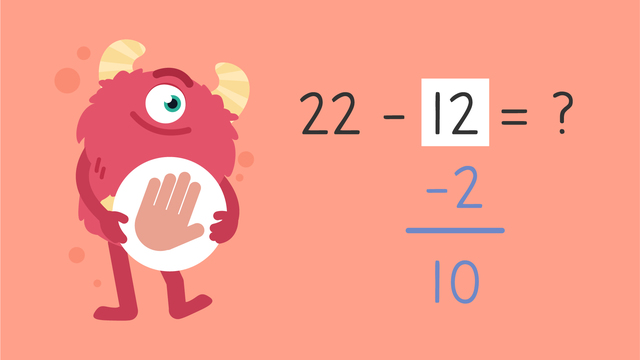# Subtraction Using Compensation — Let's Practice!Rating

Be the first to give a rating!
The authorsTeam Digital

### TranscriptSubtraction Using Compensation — Let's Practice!

Razzi says get these items ready (...) Because today we're going to practice... Subtraction using compensation. It's time to begin! Remember that compensation is something given in return for something. In math we use this with numbers. If Imani wants to subtract twelve screws from twenty-two, how many will be left over? Start by choosing which number to make friendlier. Next, solve using mental math, or your pencil and paper. Did you also get ten? Let's tackle the next problem! If Mr. Squeaks wants to subtract eighteen seeds from thirty-five, how many will he have left over? Start by choosing which number to make friendlier. Next, solve using mental math, or your pencil and paper. Did you also get seventeen? Let's tackle the next problem! If Imani wants to subtract fourteen bolts from the thirty-six, how many will be left over? Start by choosing which number to make friendlier. Next, solve using mental math, or your pencil and paper. Did you also get twenty-two ? Let's tackle the final problem! If Mr. Squeaks wants to subtract twenty-one flowers from the sixty-three, how many will he have left over? Start by choosing which number to make friendlier. Next, solve using mental math, or your pencil and paper. Did you also get forty-two? Razzi had so much fun practicing with you today! See you next time!